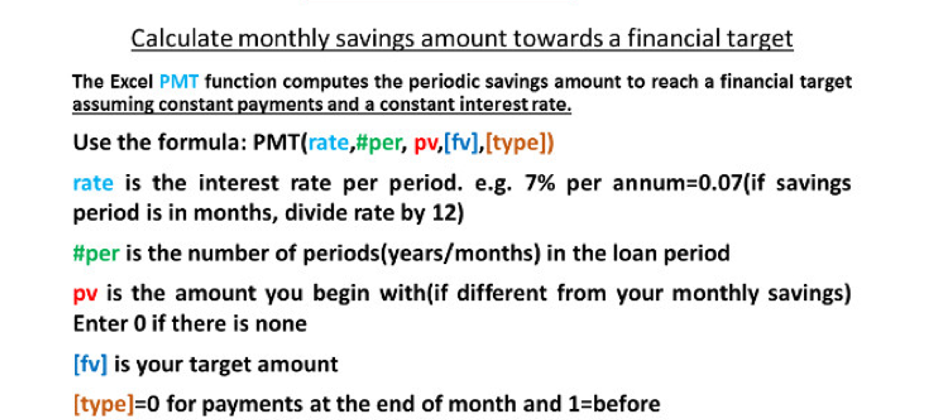top of page

# Saving Towards a Financial Target: Using Excel's PMT FormulaThe Excel PMT function is not only useful in calculating periodic payments on loans, it is also useful in calculating the periodic monthly savings amount you need to set aside to reach a financial goal. Let’s take the following example:

Question

Mr. Binfo wants to save towards his child’s education. Her daughter will need at least GH¢12,000 to make it through Primary School. Mr. Binfo wants to set aside a monthly savings amount to enable him to raise at least GH¢12,000 in the next 5 years. Mr. Binfo’s bank has offered to give him an interest of 7% p.a on the savings account he will make the monthly deposits into.

All things being equal, how much, on a monthly basis, should Mr. Binfo deposit into his savings account to enable him to raise 12,000 5 years from now?

Breaking down Mr. Binfo’s problem, we have the following inputs:

Target Sum - 12,000

Interest - 7% p.a

Period - 5 years

To determine the monthly amount, we will use the Excel PMT function. The formula requires the following inputs:

• rate is the interest rate per period. e.g. 7% per annum=0.07(if savings period is in months, divide rate by 12)

• #per is the number of periods (years/months) in the loan period (this should be consistent with the rate. If the rate is in months, the period should be in months)

• PV is the amount you begin with(if different from your monthly savings. Enter 0 if there is none. In our example, if Mr. Binfo had a beginning balance in the account already, we will use that. Otherwise, we input 0

• FV is the target amount, GH¢12,000 in our example

• [type] 0= for payments at the end of the month and 1= before the month begins. If Mr. Binfo pays before the month begins, he gets that month’s interest to be calculated as well.

Inputting our values in the formula we have:

Rate= 0.07/12

#per=60

Pv=0

Fv=12,000

Type= 1

This gives us a monthly savings amount of ¢166.64. Thus If Mr. Binfo puts aside ¢166.64 every month for the next 5 years on a savings account that gives him 7% p.a. He will raise the GH¢12,000 he needs to take care of his daughter’s Primary education.

Notes

• The PMT formula takes care of the compound interest

• You need to negate the PMT formula(-PMT). In using Excel’s Financial functions, all payments are negated and receipts are not. Our example requires payment from Mr. Binfo and hence the need to negate the entire formula. This will give us a positive figure as the formula’s result.

Enjoy practicing. Let me know how it goes.

bottom of page